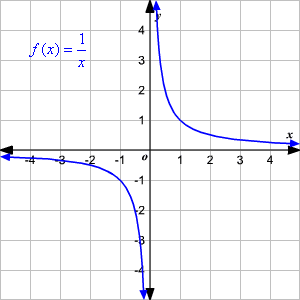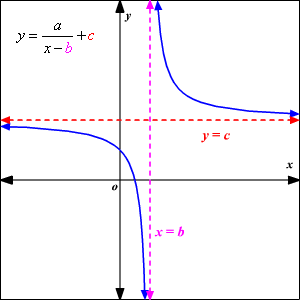# Rational Functions

A rational function is defined as the quotient of polynomials in which the denominator has a degree of at least $1$ . In other words, there must be a variable in the denominator.

The general form of a rational function is $\frac{p\left(x\right)}{q\left(x\right)}$ , where $p\left(x\right)$ and $q\left(x\right)$ are polynomials and $q\left(x\right)\ne 0$ .

Examples:

$y=\frac{3}{x},y=\frac{2x\text{\hspace{0.17em}}+\text{\hspace{0.17em}}1}{x\text{\hspace{0.17em}}+\text{\hspace{0.17em}}5},y=\frac{1}{{x}^{2}}$

The parent function of a rational function is $f\left(x\right)=\frac{1}{x}$ and the graph is a hyperbola .The domain and range is the set of all real numbers except $0$ .

$\begin{array}{l}\text{Domain:}\left\{x\text{\hspace{0.17em}}|\text{\hspace{0.17em}}x\ne 0\right\}\\ \text{Range:}\left\{y\text{\hspace{0.17em}}|\text{\hspace{0.17em}}y\ne 0\right\}\end{array}$

Excluded value

In a rational function, an excluded value is any $x$ -value that makes the function value $y$ undefined. So, these values should be excluded from the domain of the function.

For example, the excluded value of the function $y=\frac{2}{x\text{\hspace{0.17em}}+\text{\hspace{0.17em}}3}$ is –3. That is, when $x=-3$ , the value of $y$ is undefined.

So, the domain of this function is set of all real numbers except $-3$ .

Asymptotes

An asymptote is a line that the graph of the function approaches, but never touches. In the parent function $f\left(x\right)=\frac{1}{x}$ , both the $x$ - and $y$ -axes are asymptotes. The graph of the parent function will get closer and closer to but never touches the asymptotes.

A rational function in the form $y=\frac{a}{x\text{\hspace{0.17em}}-\text{\hspace{0.17em}}b}+c$ has a vertical asymptote at the excluded value, or $x=b$ , and a horizontal asymptote at $y=c$ .